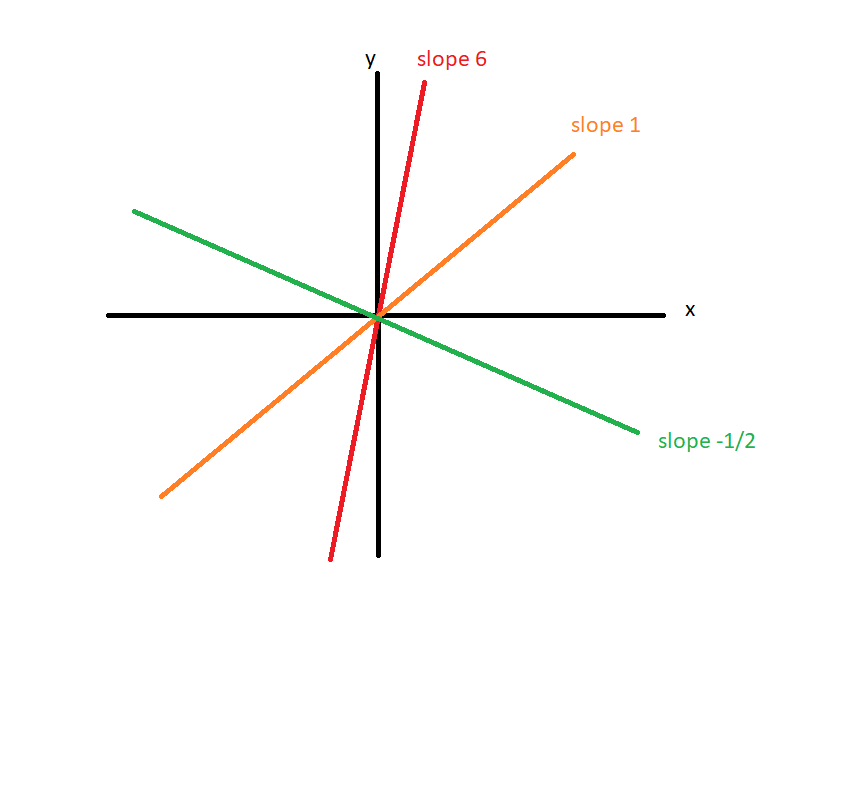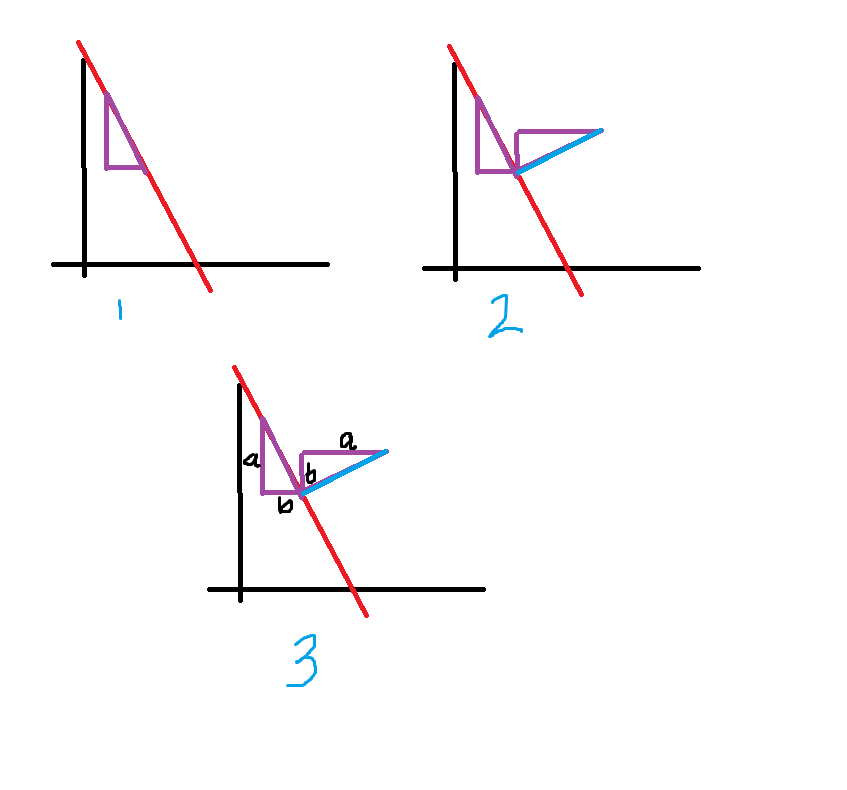# The question in Your Turn Explanation(3 of 5)

• I cannot understand the explanation of the question in Your Turn Explanation(3 of 5)

• Basically, Prof. Loh just introduced the idea of slope, which is a measure of how "slanted" a line is. Qualitatively speaking, a line with a positive slope always goes upwards when you move from left to right, and a line with negative slope always goes downwards when you move from left to right (so it makes sense that a line with slope 0 is just horizontal-- it doesn't go up or down when you move from left to right).

Also, the further away from 0 a slope is, the "steeper" it is. (So, lines with slopes 5 or -7 would be "steeper" than a line with slope 2) This diagram might help:The quantitative way of determining the slope of a line is to take two points on that line and calculate how much y increases and divide that by how much x increases between those two points. So for example, if the points are (1,2) and (5,3), then the line with those points has slope (3-2)/(5-1) = 1/4.

The main point of that specific video was to illustrate a cool fact about perpendicular lines: their slopes multiply to -1In that particular problem, the slopes were 2 and -1/2. But Prof. Loh shows how this generalizes: given any line, you can draw a right triangles whose hypotenuse is on the line (1).Then, to find a line perpendicular to the red one, you simply rotate the triangle. (2) The hypotenuse of the new triangle is a segment on the perpendicular line (if it isn't clear why, let me know!)

The above is basically what Prof. Loh did in Part 2 (with actual numbers, though). And then, to prove that in general, slopes of perpendicular lines multiply to -1, we assign variables to the legs of the purple triangle. (see 3)

The slope of the red line is (-a)/b. The slope of the blue line is b/a. (Using what we know about how to find slopes). And when you multiply the slopes: (-a)/b * b/a = -1, no matter what a and b are!

Hope that helped, and please let me know if you have further questions• Thank you, but now, I am confused about the explanation of the following question in Your Turn Explanation(3 of 5)

• I mean I cannot understand where did those big numbers come from.

And ahh, that definitely seems like an error-- the numbers probably got changed to be lower in the video and not in the explanation. The general strategy to solve is the same, just with different numbers, but I'll pass it on. Thanks!